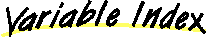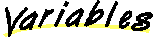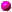`All Packages  Class Hierarchy  This Package  Previous  Next  Index`

```java.lang.Object
|
```

extends Object
implements Pigment

Author:
Daniel F. Savarese

##color1color2gradient

##Gradient()
Creates a default gradient from black to white.Gradient(Color, Color, GeometricVector)

##getColor(Point, Point, Color)
Given the origin of an object, and a point (presumably on the object) return the color (by reference) of that point assuming a gradient pigment.

##color1
```  public Color color1
```color2
```  public Color color2
```gradient
```  public GeometricVector gradient
```

##Gradient
```  public Gradient()
```
Creates a default gradient from black to white.Gradient
```  public Gradient(Color c1,
Color c2,
GeometricVector g)
```

##getColor
```  public void getColor(Point origin,
Point point,
Color colorResult)
```
Given the origin of an object, and a point (presumably on the object) return the color (by reference) of that point assuming a gradient pigment. The gradient is defined as follows. Project the point (i.e. the vector point - origin) onto the gradient vector. At the origin of the gradient vector the color is color1, and at the end of the gradient vector, the color is color2. The colors change from color1 to color2 and then again from color2 to color1, etc. as you travel along the gradient vector and beyond it's two extremes.

After projecting the point onto the gradient, the returned color is the color on the gradient at the projected point. You can do the color interpolation in various ways. This particular implementation uses a linear interpolation. I've commented out an alternate method that uses a cosine function providing wider bands near the endpoints and sharper gradation near the center.

Parameters:
origin - The origin of the object.
point - The point on the object.
colorResult - The color of the gradient at the point.

`All Packages  Class Hierarchy  This Package  Previous  Next  Index`